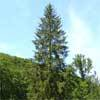#### You may also like### Baby Circle

A small circle fits between two touching circles so that all three circles touch each other and have a common tangent? What is the exact radius of the smallest circle?### Logosquares

Ten squares form regular rings either with adjacent or opposite vertices touching. Calculate the inner and outer radii of the rings that surround the squares.### Two Trees

Two trees 20 metres and 30 metres long, lean across a passageway between two vertical walls. They cross at a point 8 metres above the ground. What is the distance between the foot of the trees?

# Incircles

##### Age 16 to 18 Challenge Level:

Why do this problem?
The first two parts require only applying the idea that the tangents from an external point to a circle are equal and the radius is perpendicular to the tangent at the point of contact.

Possible approach
The first two parts could be tackled by the learners independently.

For the third part the formula for generating Pythagorean triples is needed.

Key questions
What facts do you know about tangents to circles?

What do you know about areas of triangles?

What is the formula for generating Pythagorean triples?

Possible support
Three articles: Pythagorean Triples I, Pythagorean Triples II and Picturing Pythagorean Triples.

Possible extension
The problem Pythagoras Mod 5
See the article Incircles which generalises this problem.